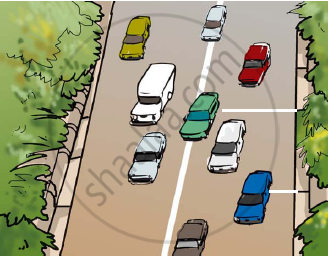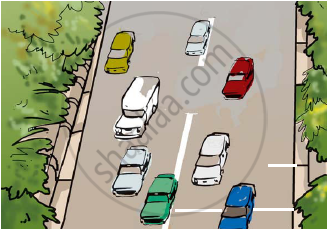Share

# Suppose the Two Photographs, Shown in Figure 1 and Figure 2, Had Been Taken at an Interval of 10 Seconds. If a Distance of 100 Metres is Shown by 1 Cm in These Photographs, Calculate the Speed of the Blue Car. - Science

Course

#### Question

Suppose the two photographs, shown in Figure 1 and Figure 2, had been taken at an interval of 10 seconds. If a distance of 100 metres is shown by 1 cm in these photographs, calculate the speed of the blue car.Figure 1 Vehicles moving in the same direction of on a roadFigure 2 Position of vehicles shown in Figure 1 after some time

#### Solution

The distance covered by the blue car (as evident from the photograph) from one white strip to another, which is measured by scale is 1.4 cm.

It is given that 1 cm is equivalent to 100 m.

Therefore, 1.4 cm is equivalent to 140 m.

Distance travelled by the car = 140 m

Time interval between the two photographs = 10 s

Speed = "Distance covered"/"Time taken" = 140/10 = 14 "m/s"

Is there an error in this question or solution?

#### APPEARS IN

NCERT Solution for Science Textbook for Class 7 (2018 (Latest))
Chapter 13: Motion and Time
Q: 11 | Page no. 157
Solution Suppose the Two Photographs, Shown in Figure 1 and Figure 2, Had Been Taken at an Interval of 10 Seconds. If a Distance of 100 Metres is Shown by 1 Cm in These Photographs, Calculate the Speed of the Blue Car. Concept: Concept of Speed.
S